# Geometric genus

(diff) ← Older revision | Latest revision (diff) | Newer revision → (diff)
A numerical invariant of non-singular algebraic varieties. In the case of algebraic curves the geometric genus becomes identical with the genus of the curve (cf. Genus of a curve). The geometric genus for algebraic surfaces was first defined from different points of view by A. Clebsch and M. Noether in the second half of the 19th century. Noether also demonstrated the birational invariance of the geometric genus. The geometric genus of a non-singular projective algebraic varietyover an algebraically closed fieldis, by definition, the dimension of the space of regular differential forms (cf. Differential form) of degree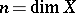. In such a case the geometric genus ofis denoted by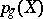. In accordance with Serre's duality theorem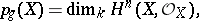where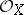is the structure sheaf of. The number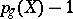coincides with the dimension of the canonical system of(cf. also Divisor). The geometric genus plays an important role in the criterium of rationality of algebraic surfaces (cf. Rational surface) and also in the general classification of algebraic surfaces. The geometric genera of birationally-isomorphic smooth projective varieties coincide.